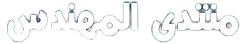# سؤال فى الماتلاب

[LEFT]asslamu alikom

How i can Solve Differential equation in matlab
?

i need to solve bessel function
in TE mode for coaxial cable[SIZE=5]

``````         [COLOR=DarkOrange]Jn'(x) Yn'(x) - Yn'(x) Jn'(X ) =0
``````

[SIZE=3][COLOR=Black]i need to get X , How
???
please if any one have an Idea about that , help me

Thanks
ur sister
eng* freska

[/color][/size]
[/color][/size]
[/left]

السلام عليكم ورحمة الله وبركاته
طبعا مهما تكون المعادلة فطريقة الحل تعتمد على استخدم ما يسمى
Symbolic Math Toolbox
وفى البداية تقومى بعمل Symbolic object لكل المتغيرات الموجودة فى المعادلة مثلا

``````x = sym('x')
a = sym('alpha')
``````

ثم تقومى بكتابة المعادلة المطلوب حلها مع وضع ال Symbolic object

ويمكنك استخدام الاوامر الخاصة الموجودة فى البرنامج
وللتعرف عليها من نفذى الامر الاتى

``````help mfunlist
``````

وستجدى مجموعة من الاوامر لحل معادلة bessel function

``````BesselI        x1,x    Bessel Function of the First Kind
BesselJ        x1,x    Bessel Function of the First Kind
BesselK        x1,x    Bessel Function of the Second Kind
BesselY        x1,x    Bessel Function of the Second Kind
``````